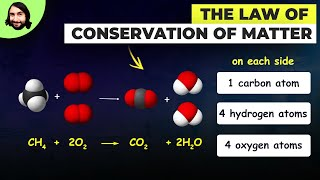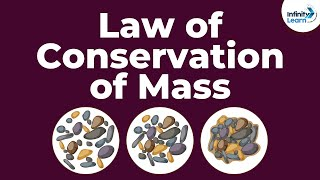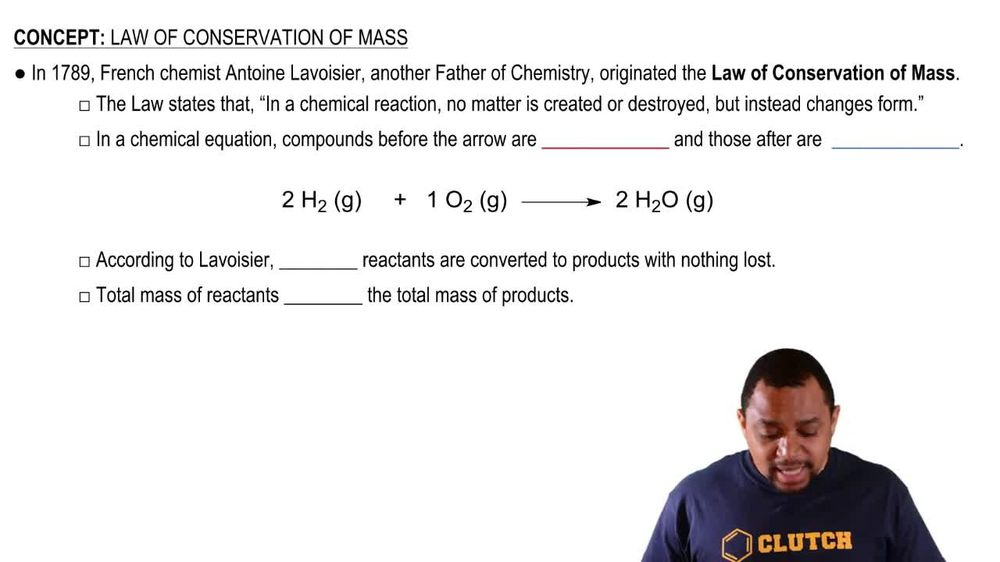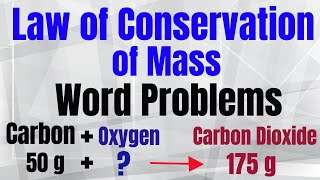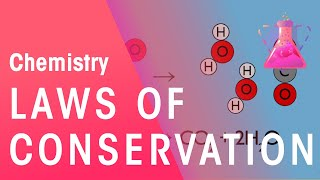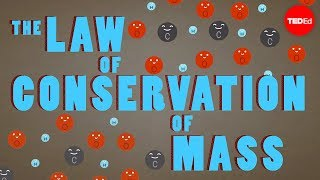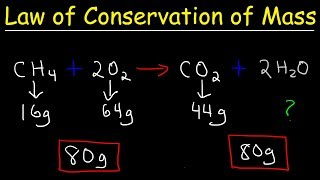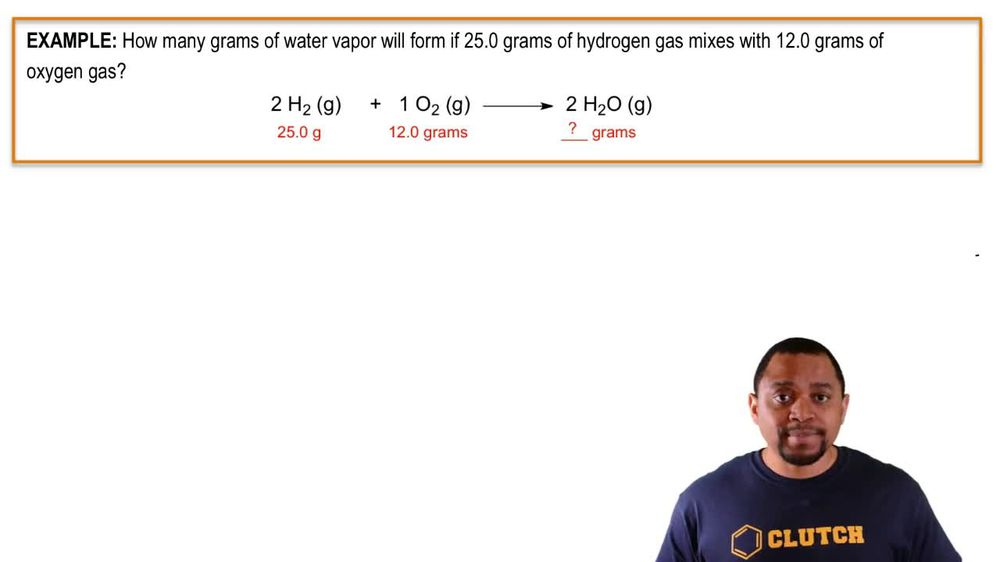Start typing, then use the up and down arrows to select an option from the list.
1. 2. Atoms & Elements2. Law of Conservation of Mass
Problem

# A sample of mercury with a mass of 114.0 g was combined with 12.8 g of oxygen gas, and the resulting reaction gave 123.1 g of mercury(II) oxide. How much oxygen was left over after the reaction was complete?

Relevant Solution2m
Play a video:
Hello everyone today, we are being given the falling problem. The reaction between 128 g of iron with 15. g of oxygen gas gave 112.4 g of iron three oxide. How much oxygen remained after the reaction was finished. So the first thing we want to note is the law of conservation of mass. Which states that in any chemical reaction the total mass of the reactant is going to equal the total mass of our products. For example, if we use one g of X, we get one g of Y. And so now we can find out our mass of our reactant. We can go ahead and add those up. So our mass of our reactant is going to include the mass of our iron plus our mass of our oxygen gas. Which is going to be 128 g for the iron plus the 15.3 g for the gas. And so that's going to give us 143.3 g. We're not done yet. We then have to find the mass of our products. So the mass of our products is going to be the mass of our iron three oxide, F. E 23 plus the mass of excess oxygen gas. And so when we do that, we get 112.4 g of that iron three oxide plus the mass of the excess 02. And so since we know that the total mass of our product products must equal the total mass of our reactant. We can go ahead and equal this to 143.3 g. And so when we subtract 112.4 g from both sides, we get 30.9 g being the mass of our excess oxygen gas. And this is our final answer. Overall, I hope this helped until next time.